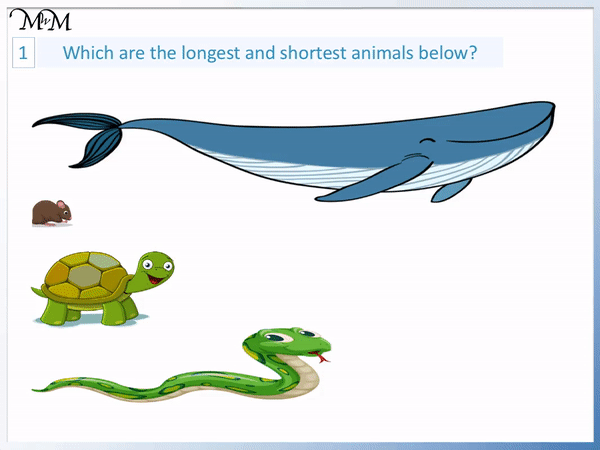# Comparing Lengths as Longest, Shortest and Tallest

Comparing Lengths as Longest, Shortest and Tallest• The length of an object is its biggest distance from one end to the other.
• We can compare the length of two objects by lining them up at one end.
• We can line the start of the snake up with the start of the tortoise.
• The distance from one end of the snake to the other is bigger than the distance from one end of the tortoise to the other.
• We say that the snake is long compared to the tortoise.
• We say that the tortoise is short compared to the snake.• The height of an object is measured vertically, directly from the ground upwards.
• The height of an object is its length upwards.
• The tree is longer than the flower but with height we use the word tall.
• Compared to the flower, the tree is tall.
• The flower is short compared to the tree.

Length is the largest distance from one side of an object to the other.

We use the words short and long to describe length but the words short and tall to describe height.

• Something is the shortest if it is described as short compared to everything else.
• Something is the longest if it is described as long compared to everything else.
• The whale is longer than all of the other animals and so the whale is the longest.
• The mouse is shorter than all of the other animals and so the mouse is the shortest.# How do we Compare Lengths Using the Words Shortest, Tallest and Longest?

Length is the biggest distance from one end of an object to the other.

In this lesson we are comparing objects to see which is the longest and shortest.

In the first example below, we have a tortoise and a snake.When teaching comparing length, it can help to make sure that the two objects are lined up to start at the same place. This makes it much easier to visualise and understand this concept if it is new.

The snake’s length is bigger than the tortoise’s length since it is bigger from one end to the other.

We say that the snake is long compared to the tortoise. Or we say that the snake is longer.

We say that the tortoise is short compared to the snake.

We can say that the tortoise is shorter than the snake.

Remember that both long and short are comparative words. Whilst the snake is long compared to the tortoise, the snake can still be short compared to something longer.

Here is an example of comparing the lengths of three animals.Remember that to compare their lengths, it is easiest to line the objects up first.

We can see that the horse is long compared to both the dog and the tortoise.

If something is longer than everything else, we say it is the longest.

The horse is the longest animal.

If something is shorter than everything else, we say it is the shortest.

The tortoise is the shortest animal.

The concept of height is very similar to length. Height is the length of an object upwards, its total distance from the ground up.

In the example of comparing heights below, we have a tree and a flower.Just like with length, the object with the smallest distance is called short.

The flower has a smaller height compared to the tree and so it is short.

The tree is long compared to the flower, however we use the word tall instead of long when talking about height.

In the example below, we have 5 plants that we will compare the heights of.This plant is shorter than all of the others and so, it is the shortest.

The stalk on the right is taller than all of the other plants and so it is the tallest.

In the example problem below, we are asked to find the longest and shortest animals.The whale is long compared to every other animal and so, it is the longest.

The mouse is short compared to every other animal and so, it is the shortest.

In the example below, we are comparing heights to find the shortest and tallest plants.The tree on the left is taller than all of the other plants and so it is the tallest.

The plant on the right is short compared to every other plant and so, it is the shortest.Now try our lesson on Measuring Centimetres Using a Ruler where we learn how to use a ruler to measure the length of objects.